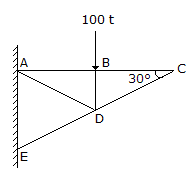# Civil Engineering - Theory of Structures - Discussion

### Discussion :: Theory of Structures - Section 3 (Q.No.4)

4.

In the truss shown in given figure the force in member DC is[A]. 100 t compressive [B]. 100 t tensile [C]. zero [D]. indeterminate

Explanation:

No answer description available for this question.

 Sanyogita said: (Feb 11, 2015) First consider joint C then find out forces fbc & fcd by using summation fx & fy then get answer zero.

 Sophia said: (Feb 12, 2015) How did you come around zero?

 Alagarbrindha said: (Mar 27, 2020) Take joint C, Summation of vertical force as zero, Force in DC * cos 30 = 0. Hence force in DC = 0.